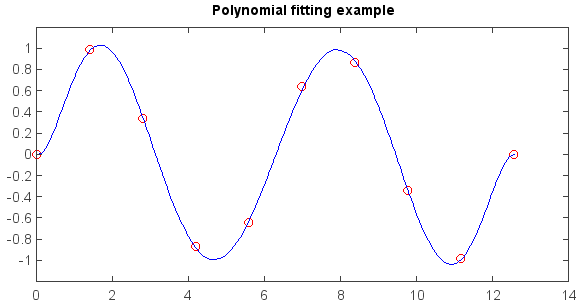# polyval¶

numeric.fitting.polyval(p, x)

Evaluate a polynomial at specific values.

If p is of length N, this function returns the value:

p*x**(N-1) + p*x**(N-2) + … + p[N-2]*x + p[N-1]

If x is a sequence, then p(x) is returned for each element of x. If x is another polynomial then the composite polynomial p(x(t)) is returned.

Parameters
• p – (array_like) 1D array of polynomial coefficients (including coefficients equal to zero) from highest degree to the constant term.

• x – (array_like) A number, an array of numbers, or an instance of poly1d, at which to evaluate p.

Returns

Polynomial value

Examples:

```from mipylib.numeric import fitting

x = linspace(0, 4*pi, 10)
y = sin(x)

#Plot data points
plot(x, y, 'ro', fill=False, size=1)

#Use polyfit to fit a 7th-degree polynomial to the points
r = fitting.polyfit(x, y, 7)

#Plot fitting line
xx = linspace(0, 4*pi, 100)
p = r
yy = fitting.polyval(p, xx)
plot(xx, yy, '-b')
title('Polynomial fitting example')
```